# Equivalent equation

Which equation, when solved, results in a different value of x than the other three?

A.
$-\frac{7}{8}x-\frac{3}{4}=20$

Negative 7/8 x minus three-fourths = 20

B.
$\frac{3}{4}+\frac{7}{8}x=-20$

Three-fourths + 7/8 x = negative 20

C.
$-7\frac{1}{8}x-3\mathrm{/}4=20$

Negative 7 ( 1/8 ) x minus three-fourths = 20

D.
$-\frac{7}{8}\left(-\frac{8}{7}x\right)-\frac{3}{4}=20\left(-\frac{8}{7}\right)$

Negative 7/8 (Negative 8/7 ) x minus three-fourths = 20 (Negative 8/7 )

Result

w = (Correct answer is: D)### Step-by-step explanation: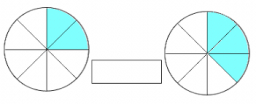Did you find an error or inaccuracy? Feel free to write us. Thank you!Tips to related online calculators
Need help to calculate sum, simplify or multiply fractions? Try our fraction calculator.
Do you have a linear equation or system of equations and looking for its solution? Or do you have a quadratic equation?

## Related math problems and questions:

• Equation with fractionsWhat is another way to write the equation 7/8 x + three-fourths = negative 6?
• Patel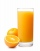Patel squeezed oranges so that his family could have fresh-squeezed juice for breakfast. He squeezed 4/17 cups from the first orange, 3/10 cups from the second orange, StartFraction 9 over 20 E
• Equivalent expressionPablo rewrites the expression 8y-24 as 8(y-3). Hana rewrites 8y-24 as 2(4y-12). Are Pablo’s and Hana’s expressions each equivalent to 8y-24? Explain your reasoning.
• StudentsStudents solve word problems. They received 3 points for each correctly solved task. Four points were deducted for a task that was not solved or incorrectly solved. Tomas solved a total of 15 tasks and scored 24 points. How many tasks did he solve correct
• BoatsThree-quarters of boats are white, 1/7 are blue and 9 are red. How many boats do we have?
• Fraction expressionWhich expression is equivalent to : minus 9 minus left parenthesis minus 4 start fraction 1 divided by 3 end fraction right parenthesis
• Equivalent fractionsAre these two fractions equivalent -4/9 and 11/15?
• Equation with fractionsWhat is the simplified form of the equation fraction 4 over 5 n minus fraction 1 over 5 equals fraction 2 over 5 n? n = -2 n = 4 n = fraction 1 over 2
• The math testThe math test contains 20 problems. For each correctly solved problem, the solver gets 3 points, for each incorrectly solved or unsolved problem, 2 points are deducted. Ondrej got 25 points. How many problems did he solve correctly?
• Fraction unknowns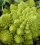Divide of fractions with unknowns: Fraction 1: The quantity x squared plus 6 times x plus 9 over the quantity x minus 1. Fraction 2: the quantity x squared minus 9 over the quantity x squared minus 2 times x plus 1.  Find Fraction 1 over Fraction 2.
• Simple equation 324 = n • 27, solve for n
• Equivalent fractions 2Write the equivalent multiplication expression. 2 1/6÷3/4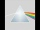Please determine the solvability conditions of the equation, solve the equation and perform the test: x divided by x squared minus 2x plus1 the whole minus x + 3 divided by x squared minus 1 this is equal to 0: x/(x2-2x+1) - (x+3)/( x2-1) = 02x + 3 + 7x = – 24, what is the value of x?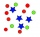Which is an equivalent ratio for 12:36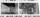Negative dimensions are 36mm and 28mm. What will be the photo size in the 21:4 ratio?What is the value of x in the proportion 2 and one-fourth over x = 1 and one-half over 3 and three-fifths? 2 and two-fifths 5 and two-fifths 8 and 1 over 10 12 and 3 over 20